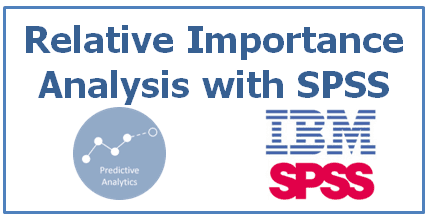# Relative Importance (Weight) Analysis with SPSS

Relative Weight (Importance) Analysis

Relative Weight Analysis is a useful technique to calculate the relative importance of predictors (independent variables) when independent variables are correlated to each other. It is an alternative to multiple regression technique and it addresses multicollinearity problem and also helps to calculate the importance rank of variables. It helps to answer " Which variable is the most important and rank variables based on their contribution to R-Square".Relative Importance Analysis in SPSS

Background

When independent variables are correlated, it is difficult to determine the correct prediction power of each variable. Hence, it is difficult to rank them as we are unable to estimate coefficients correctly. Statistically, multicollinearity can increase the standard error of the coefficient estimates and make the estimates very sensitive to minor changes in the model. It means the coefficients are biased and difficult to interpret.

How it works

It creates a set of new independent variables that are the maximally related to the original independent variables but are uncorrelated to each other. Because these new transformed independent variables are uncorrelated to each other, the dependent variable can be regressed onto this new set of independent variables producing a series of standardized regression coefficients.

Calculation Steps
1. Compute correlation matrix between independent variables
2. Calculate Eigenvector and Eigenvalues on the above correlation matrix
3. Calculate diagonal matrix of eigenvalue and then take square root of the diagonal matrix
4. Calculate matrix multiplication of eigenvector, matrix in step 3 and Transpose of Eigenvector
5. Square the above matrix
6. To calculate the partial effect of each independent variable on dependent variable, calculate matrix multiplication of [Inverse of matrix in step 4] and correlation matrix [between dependent and independent variables (i.e. 1 X 1 matrix)]
7. To calculate R-Square, sum the above matrix (Step 6 matrix)
8. To calculate raw relative weights, calculate matrix multiplication of [matrix in step 5] and [Square of matrix in step 6]
9. To calculate raw relative weights as percentage of R-Square, divide raw relative weights by r-square and then multiply it by 100.

SPSS Code : Relative Weight (Importance) Analysis
***************************************************
********** RELATIVE WEIGHT ANALYSIS ************
***********Author : Deepanshu Bhalla*******************
****************************************************

*Specify a path where INPUT DATA FILE is saved.

*Specify a path where you wish OUTPUT files to be saved.

*Define Independent Variable names.
DEFINE Ivars ( )
var1 var2 var3
!ENDDEFINE .

*Define Dependent Variable name.
DEFINE Target ( )
Churn
!ENDDEFINE .

*Define VARIABLE LABELING for Independent variables.
*Order of variable labeling and independent variables must be same.
*Space in labels should not be used, rather words separated by"_".
DEFINE LABELING ( )
Interest_Rate
Renewed_PCT
Account_No
!ENDDEFINE.

GET FILE = 'DataFile'.

CORRELATIONS
/VARIABLES= Target Ivars
/MISSING=PAIRWISE
/MATRIX = OUT (Corr.sav).

oms
/select tables
/if commands =['Regression'] SUBTYPES=['Coefficients']
/destination format =SAV  outfile ='Betas.sav'
/columns sequence =[RALL CALL LALL].

regression
/dependent Target
/method= enter Ivars.
omsend.

GET FILE='Betas.sav'.

FLIP ALL.

COMPUTE var6=INDEX(CASE_LBL, '_Beta').
RECODE var6 (1 THRU HIGHEST=1) (else=0).
SELECT IF var6=1.
EXECUTE .

DELETE VARIABLES CASE_LBL VAR6.

SAVE Outfile = 'Directory\Coefficients.sav'
/ RENAME = (var001=Coefficients).

GET FILE = corr.sav .
SELECT IF rowtype_ = 'CORR' .
EXECUTE.

matrix.

MGET / FILE = 'Corr.sav'
/ TYPE = CORR.

COMPUTE R = CR.

COMPUTE N = NCOL(R).
COMPUTE RXX = R(2:N,2:N).
COMPUTE RXY = R(2:N,1).
CALL EIGEN(RXX,EVEC,EV).
COMPUTE D = MDIAG(EV).
COMPUTE DELTA = SQRT(D).
COMPUTE LAMBDA = EVEC * DELTA * T(EVEC).
COMPUTE LAMBDASQ = LAMBDA &**2.
COMPUTE BETA1 = INV(LAMBDA) * RXY.
COMPUTE RSQUARE = CSSQ(BETA1).
COMPUTE RAWWGT = LAMBDASQ * BETA1 &**2.
COMPUTE IMPORT = (RAWWGT &/ RSQUARE) * 100.

PRINT RSQUARE /FORMAT=F8.8.
PRINT RAWWGT /FORMAT=F8.8
/TITLE = "Raw Relative Weights" .
PRINT IMPORT /FORMAT=PCT8.8
/TITLE = "Relative Weights as Percentage of R-square" .

SAVE RSQUARE
/OUTFILE='RSQ.sav'.
SAVE RAWWGT
/OUTFILE='Raw.sav'.
SAVE IMPORT
/OUTFILE='Relative.sav'.
END MATRIX.

INPUT PROGRAM.
NUMERIC LABELING (F25).
LOOP #=1 TO 1.
END CASE.
END LOOP.
END FILE.
END INPUT PROGRAM.
FLIP.

SAVE OUTFILE = 'Labeling.sav'
/ DROP VAR001
/ RENAME (CASE_LBL=Categories).

MATCH FILES FILE ='Labeling.sav'
/ FILE = 'Raw.sav'
/ Rename = (COL1 = RAW_RELATIVE)
/ FILE = 'Relative.sav'
/ Rename = (COL1 = PERCENT_RSQUARE)
/ FILE = 'Directory\Coefficients.sav'
/ FILE = 'RSQ.sav'
/ Rename = (COL1 = RSQUARE).

FORMATS  RAW_RELATIVE TO RSQUARE (F8.6).

SAVE TRANSLATE OUTFILE='Directory\Final_Output.xls'
/TYPE=XLS
/VERSION=8
/REPLACE
/FIELDNAMES
/CELLS=VALUES.
EXECUTE.Share
Related PostsDeepanshu founded ListenData with a simple objective - Make analytics easy to understand and follow. He has over 10 years of experience in data science. During his tenure, he has worked with global clients in various domains like Banking, Insurance, Private Equity, Telecom and Human Resource.

9 Responses to "Relative Importance (Weight) Analysis with SPSS"
1.Thanks for this interesting post.
However I'm experiencing some difficulties in following some steps, particularly steps 4 and 6
Could you please post an example of processing, say a 3 x 3 correlation matrix ?

2.This is excellent!

3.KlaZe it 13roSki

4.This comment has been removed by a blog administrator.

5.Thanks a lot. This is very helpful. May I know how to revise the syntax if the raw data is a correlation matrix?

6.Thank you for the code. Worked really well. Do you have a reference on interpretation of output?

7.Thanks for the above, I tried to use the syntax on spss 20 but produces error. Any advice?

8.How I can calculate RII for multiple variables by using SPSS means for clients, contractor consultant and other factors and there are many questions under above listed parties

9.This comment has been removed by a blog administrator.

Next → ← Prev
Love this post? Support Us!